Most of the Mathematical Puzzles published on this website are represented as Picture Puzzle Images. These Maths Puzzles can be used as worksheets in schools. These Maths Puzzles are in Printable format where each individual picture puzzle image can be downloaded and printed for solving. Anyway here are more Maths Picture Puzzles to challenge your mind.
In each of these Maths Picture Puzzles, there are four groups of numbers. Each group is having five numbers. These five numbers in each group are related to each other by a Mathematical pattern. One number is missing in one of the groups. Your challenge is to find this mathematical pattern in each Maths Picture Puzzle and find the missing number which will replace the question mark.

Answers to these Maths Picture Puzzles are given at the end.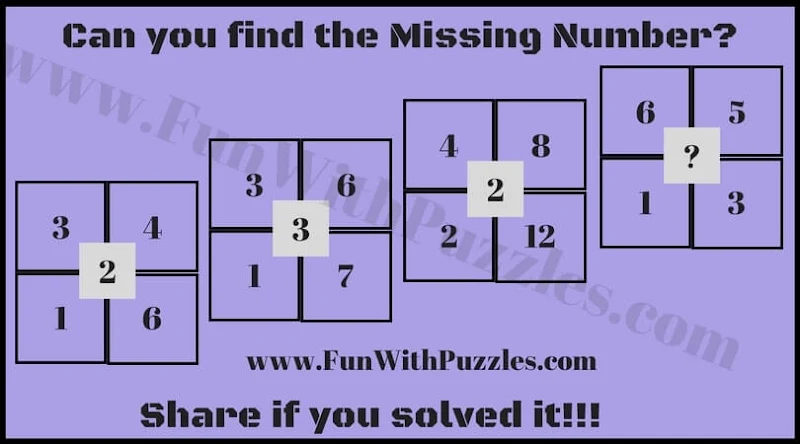1. Can you find the missing number?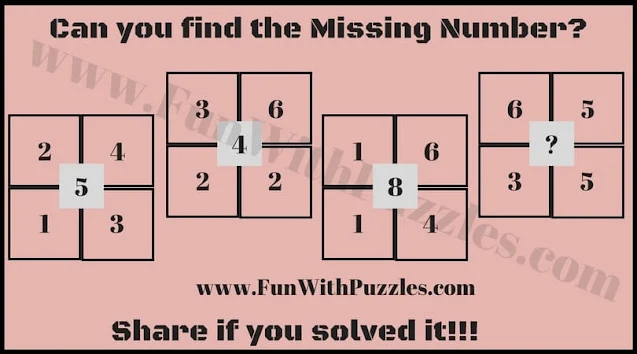2. Can you solve it for the missing number?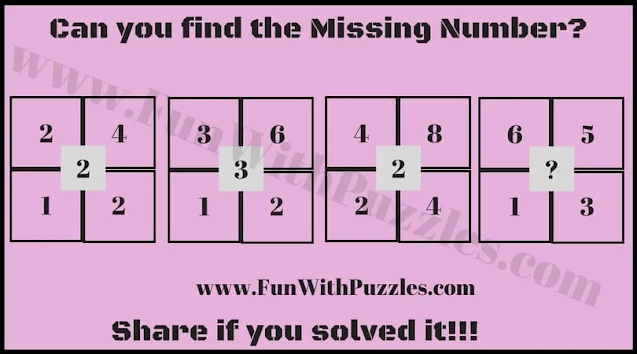3. Which Number will replace the question mark?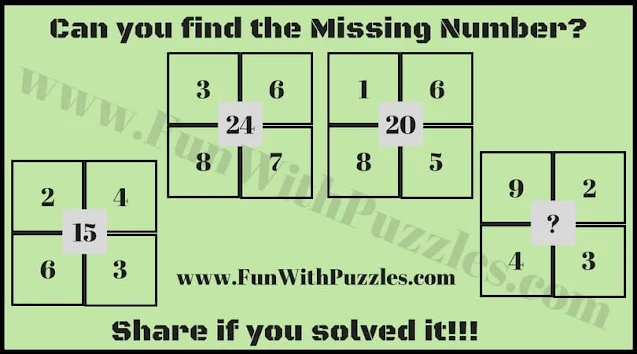4. Solve this Maths Brain Teaser and find the missing number?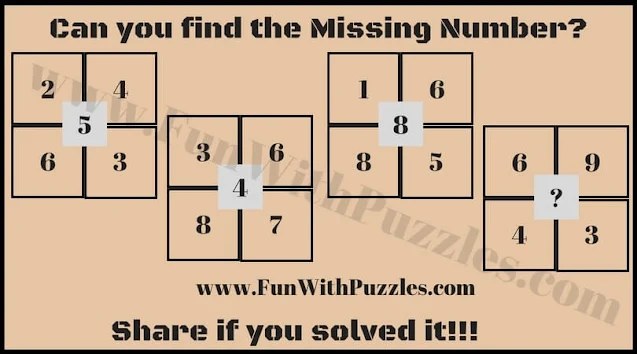5. What will come at the question mark?

You can have fun solving with more Maths Puzzles as mentioned below
More Maths Brain Teasers to challenge your mind

1. Matchstick Maths Puzzles: In these Maths Picture Puzzles, one has to correct the given equations by moving exactly one matchstick.

2. Kids Maths Equations Maths Puzzles: These are the easy maths equations puzzles in which variables are replaced with shapes and your challenge is to solve these maths equations to find the values of these shapes.

3. Brain Teasers and Riddles IQ Test: This is the Brain IQ Test to challenge your brain. This IQ test contains many different types of Maths Picture Puzzles.

4. Math Brain Teasers for Kids with Answers and Explanations: It contains very interesting Maths Puzzles in which your challenge is to solve the equations for the given symbols.

Answers to these Maths Picture Puzzles are given below

2) 5

3) 5

4) 18

5) 4